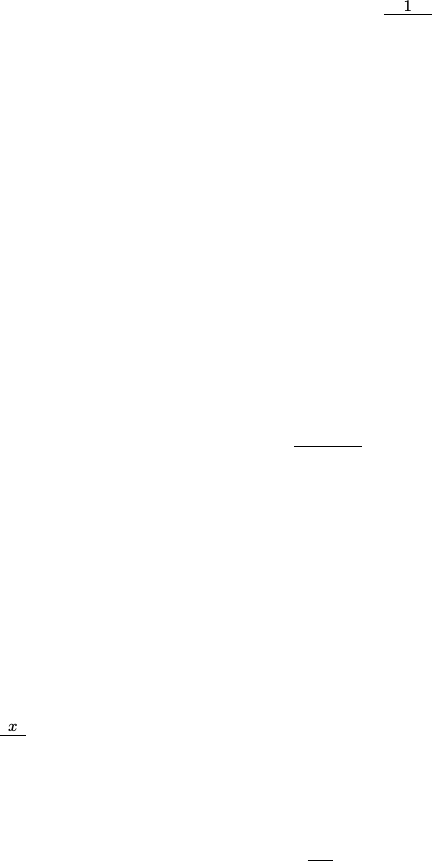# AS 5102 Study Guide - Quiz Guide: Survival Analysis, Life Table, Givet

5 views6 pagesTEMPLE UNIVERSITY
AS 3501 ACTUARIAL MODELING I
PROBLEM SET CH.2&3, LM CH.12 SOLUTION
Note: The solutions below may only provide the key steps for certain questions.
1. LTAM Sample Qs: see the solutions posted by SOA.
2. LTAM Written Sample Qs: see the solutions posted by SOA.
3. MLC Sample Qs: see the solutions posted by SOA.
4. MLC Written Sample Qs: see the solutions posted by SOA.
5. Textbook Exercises: see the answers in the textbook.
6. The force of mortality for a survival model is given by µx=1
110x,0x < 110.
(a) Find out f0(t)and S0(t).
(b) What is the limiting age ωfor this model?
(c) Assess the suitability of this survival model for human mortality.
Solution:
(a)
S0(t) = tp0
=eRt
0µrdr
=eRt
01/(110r)dr
=eln(110r)|t
0
=110 t
110
for 0t110. Thus,
f0(t) = µt·tp0= 1/110
for 0< t < 110.
(b) ω= 110 since S0(t)decreases to 0 when t= 110.
(c) First, the model assumes death occurring uniformly (not depending on age), which is not true for
human mortality. Secondly, the limiting age being 110 is also not a realistic assumption.
7. Given that µx=x
100 for x0, calculate 20|10q5.
Solution: Since 20|10q5=20p530p5, we would need to obtain tp5ﬁrst.
tp5=eRt
0µ5+rdr
=eRt
0
5+r
100 dr
=e0.01(5r+0.5r2)|t
0
=e0.05(t+0.1t2).
Unlock document

This preview shows pages 1-2 of the document.
Unlock all 6 pages and 3 million more documents.It follows that
20|10q5=20p530p5=e0.05(20+0.1·202)e0.05(30+0.1·302)= 0.0473
8. For a population which contains equal numbers of males and females at birth:
(i) The force of mortality for females, µf
x(t) = 0.08,x0
(ii) The force of mortality for males, µm
x(t) = 0.10,x0
For this population, calculate
(a) q50
(b) ˚e0
(c) ˚e20 and ˚e20:10
Solution: Note that the force of mortalities for males and females are different, which implies that
the percentage weights of males and females in the total population are changing by time! In this
question, they have equal weights only at birth. It would be easier if we transfer age xto age 0. For a
constant force of mortality, we have
Sf
0(t) = e0.08t, and Sm
0(t) = e0.1t,
for female and male, respectively. Hence, for a randomly picked newborn from this population, one
concludes that
S0(t) = 0.5Sf
0(t) + 0.5Sm
0(t) = 0.5(e0.08t+e0.1t).
(a)
q50 = 1 S0(51)
S0(50)
= 1 0.5(e0.08·51 +e0.1·51)
0.5(e0.08·50 +e0.1·50)
= 0.0818
(b)
˚e0=Z
0
S0(t)dt
=Z
0
0.5(e0.08t+e0.1t)dt
= 0.51
0.08 +1
0.1
= 11.25
(c)
˚e20 =Z
0
S0(t+ 20)
S0(20) dt
=0.5
S0(20) Z
0
(e0.08(t+20) +e0.1(t+20))dt
=1
e1.6+e2e1.6
0.08 +e2
0.1
= 11.4967
Unlock document

This preview shows pages 1-2 of the document.
Unlock all 6 pages and 3 million more documents.